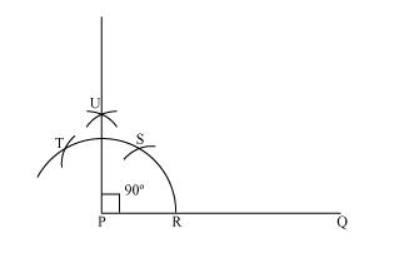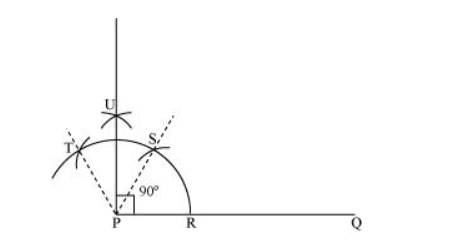# Construct an angle of 90° at the initial point of a given ray and justify the construction.

Question. Construct an angle of 90° at the initial point of a given ray and justify the construction.

Solution:

The below given steps will be followed to construct an angle of 90°.

(i) Take the given ray PQ. Draw an arc of some radius taking point P as its centre, which intersects PQ at R.

(ii) Taking R as centre and with the same radius as before, draw an arc intersecting the previously drawn arc at S.

(iii) Taking S as centre and with the same radius as before, draw an arc intersecting the arc at T (see figure).

(iv) Taking S and T as centre, draw an arc of same radius to intersect each other at U.

(v) Join PU, which is the required ray making 90° with the given ray PQ.Justification of Construction:

We can justify the construction, if we can prove $\angle U P Q=90^{\circ}$.

For this, join PS and PT.We have, $\angle S P Q=\angle T P S=60^{\circ} .$ In (iii) and (iv) steps of this construction, $P U$ was drawn as the bisector of $\angle T P S$.

$\therefore \angle \mathrm{UPS}=\frac{1}{2} \angle \mathrm{TPS}=\frac{1}{2} \times 60^{\circ}=30^{\circ}$

Also, $\angle U P Q=\angle S P Q+\angle U P S$

$=60^{\circ}+30^{\circ}$

$=90^{\circ}$